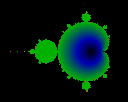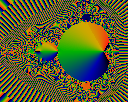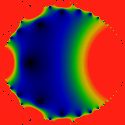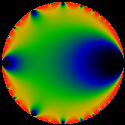An updated, revised, and more readable version of this page can be found in the math section of this website.

# Spectral Analysis of Mandelbrot Interior

The term 'spectral' or 'characteristic' analysis refers to a way of understanding the behavior of an infinite sequence by examining the behavior of various series built up from the sequence. It is occasionally used to examine the eigenvalues of infinite-dimensional representations. In particular, this technology is useful when dealing with divergent or non-convergent series. The basic idea consists of examining the analytic properties of a different, 'regulated', series.

Consider the sequence {a0, a1, a2,...} Then, for small and positive t, construct the sum S(t) = Sumn=0inf an exp (-tn) We call this sum the 'regulated' series. For large class of reasonably behaved series {an} this sum is finite for all positive values of t. (By 'reasonably behaved' we mean a sequence where an doesn't get exponentially large with increasing n; that is, one where the sum converges.). The spectral analysis consists of exploring the behavior of the sum in the limit of t->0. Depending on the series, it may diverge. For example, if we take all an to be one, the sum N(t) = Sumn=0inf exp (-tn) diverges as 1/t, while Sumn=0inf n exp (-tn) diverges as 1/t2. We can understand the series better by understanding the type of divergences it has.

Another common regulator is the (Reimann) zeta regulator: C(s) = Sumn=0inf an / sn which is explored in the limit of s->1. Note that S(t) can be derived from C(s), and v.v., through analytic means. In particular, poles in the complex s-plane map to powers of t; if C(1) is finite, then so is S(0) and v.v. However, S(t) is far easier to deal with computationally than C(s) is. In fact, to further simplify the numerical calculations, we'll use the regulator exp (-t2n2), which converges a good bit faster. It also can be analytically related to the other regulators.

This page is devoted to the application of these sums to the Mandelbrot series: zn+1 = zn2+c. Let us then consider S(t) = Sumn=0inf zn exp (-t2n2) For most of the interior of the Mandelbrot set, this sum diverges as 1/t. To render an image of this, let N(t) = Sumn=0inf exp (-t2n2) which also diverges as 1/t. We use this to show the divergence: the image below shows limt->0 |S(t)|/N(t) where |S(t)| is just the modulus of S(t); that is, |S(t)| = sqrt ((Re S(t))2 + (Im S(t))2)The color green represents a value of 0.5. Thus, it seems that the limit has a simple zero at Re c = Im c = 0. The sum is exactly equal to 1/2 in the largest bud on the left, and approximately equal to 1/2 in the other buds. Below, we will provide an exact expression for the divergent term throughout the interior.

Its somewhat more interesting to look at a related sum, the sum over second derivatives of z w.r.t. c. If we write the iterated Mandelbrot equation as so: zn+1 = zn2 + c, then, realizing that it is parameterized by c, we can take its derivative: z'n+1 = dzn+1/dc = 2 z'n zn + 1. The second derivative follows: z''n+1 = d2zn+1/dc2 = 2 (z''n zn + z'n2). Note that zn, z'n and z''n are well defined for all values of c and finite n: they are entire functions, 'merely' polynomials in c. We then define the sum: P(t) = Sumn=0inf z''n exp (-t2n2). Note that P(t) = d2 S(t) / dc2 holds for all positive t. P(t) also diverges as 1/t. The image below shows limt->0 |P(t)| / N(t)Red denotes any value equal or greater than 1. We can give an exact expression for limt->0 |P(t)| / N(t) in the main body. It is limt->0 |P(t)| / N(t) = 1/( 4 |1/4 - c|3/2 ) (It is numerically straightforward to verify that this expression is exact to between 6 and 10 decimal places. See the section 'numerical hints' below for a greater discussion). The value of this limit in the largest bud to the left is precisely zero over the entire bud. For the next smallest buds (at the top, bottom, and the second to the left), the value seems to be uniformly 1/30 across the whole bud, although there does seem to be a slight gradation which is hard to distinguish from numerical errors. By looking at this image, we can see that this limit seems to take on other, constant, values in the progressively smaller buds. The color scheme here has black <= 0.0, blue ~= 0.2, green ~= 0.5, yellow ~= 0.75, red >= 1.0. If the values were indeed constant over the smaller buds, this would have some interesting implications on the limit-cycles for these buds, as discussed below.

The next image shows the phase of P(t) in the limit of t->0.That is, it shows limt->0 arctan (Im P(t) / Re P(t)). The color scheme is such that black=-pi, green=0, red=+pi. Combining what we've learned from this and the previous image, we conclude that the divergent part of P(t) is precisely 1/( 4 (1/4 - c)3/2 )

Since P(t) = d2 S(t) / dc2, we can integrate to find the divergent part of S(t). We find that limt->0 |S(t)|/N(t) = A(c) = 1/2 - (1/4 - c) 1/2. Note that |A(c)|=1/2 on the cardiod: we can easily verify that |A (eip/2 - e2ip/4) | = 1/2 for all values of p. Next, we note that A(c) is a solution to the Mandelbrot equation: A(c) = A(c)2 + c. Indeed, this should not be a surprise: The divergent term of the sum S(t) is in effect the average over over all values of zn. Inside the main bulb, we have lim n->inf zn = A(c), and so of necessity, the leading divergence of the sum must be A(c). Similarly, in the large bud on the left, zn converges to a two-cycle, with zn -> -1/2 + i sqrt (c + 3/4) and zn+1 -> -1/2 - i sqrt (c + 3/4) . The average of these two values is -1/2, and so we can trivially deduce that limt->0 S(t)/N(t) = -1/2 and that limt->0 |S(t)|/N(t) = 1/2 which exactly matches our numerical results. In other buds, the sequence converges to m-cycles. Thus, in other buds, the divergent term will be the average over these m values of the limit cycle. Provided one can calculate this average, then one has an exact expression for the divergences of S(t).

Let us now turn to the finite remainders. By subtracting away the divergent pieces, we are essentially subtracting away the contribution of the asymptotic limit cycle. The remaining finite parts indicate how the asymptotic behaviour is approched. If the finite part is large, this indicates that the iteration took a long time to approach the asymptotic limit. If the finite part is small, then the series converged to its limit cycle quickly.This image shows limt->0 |S(t)|- A(c) N(t) . (We take A(c) to be 1/2 in the largest bud). The color ramp has been scaled by -1: i.e. black = 0, green ~= -0.5, red <= -1.0. There appear to be a number of poles arrayed along the perimeter.

Lets take an up-close look at the large bud.The sum P(t) doesn't have a divergent piece in the large bud. This image shows limt->0 |P(t)| for the area Re c = [-1.25, -0.75]. As can be seen, it has a much more complicated structure. There seem to be poles located where-ever some horns come together, and sequences of zeros inside the bud. The poles all seem to have positive residues. The color ramp has been scaled by ten: i.e. black = 0, green ~= 5, red >= 10.

Below is the finite part of S(t) in the largest bud.The image below shows limt->0 |S(t)| - 0.5*N(t) for the area Re c = [-1.3, -0.7]. It seems to have a simple structure, with a pole at c=(-0.75,0) and a zero at c=(-1.25,0). Looking more closely, one seems to see zero's arrayed along the perimeter. In fact, we can expect a rather complex structure: after all, P(t) = d2 S(t) / dc2 is an exact identity for any positive t, and we saw that the structure of P(t) is quite complex. The color ramp has been scaled by -1: i.e. black = 0, green ~= -0.5, red <= -1.0.

The image below shows the finite piece in the main bulb, after the divergent piece has been subtracted.That is, it shows limt->0 |P(t)| - N(t) / ( 4 |1/4 - c|3/2 ) It appears to have dipoles (saddles) arrayed along the perimeter. There don't seem to be any simple zeros. The color scheme has been adjusted so that black <= -10, green ~= 0 and red >= 10. These (multi-)poles visually indicate something that is commonly known: the series has a hard time converging near the tips of the horns.

Visually, this term appears to be some sort of q-series (q-pochhammer symbol) or Dedekind eta, which is immediately obvious if we compare to a figure of the Dedekind eta, below.This image shows the Dedekind eta. The visual similarity is remarkable. In particular, this helps confirm the modular group symmetry of the Mandelbrot set in a rather direct way, and gives us a pretty good idea of what conformal map is needed to establish an explicit symmetry. The Dedekind eta shows up in the theory of modular forms, which has seen a lot of activity due to thier connection to many very interesting mathematical topics. Please see the math section of this website for a detailed exposition of this relationship.

## Numerical Hints

Note that the series explored on these pages can be slow to converge, especially near the 'horns' of the Mandelbrot set. We can improve things numerically a bit with a trick to extrapolate to t=0. We do this by working with a Taylor expansion of the regulator about finite t, and performing sums with that. The sums can then be recombined in the Taylor expansion, evaluated at t=0. This technique helps hasten convergence. Working to the second derivative seems sufficient.

Note that it is wise to double-check one's work against the simplest sums. This is because working with the Taylors series requires one to keep a half-dozen or more sums, and to properly combine first and second derivatives of square-roots, products, and ratios. Easy enough on paper, but still prone to algebraic errors or typing errors when writing the code.

Once one has working expressions, it is fairly straightforward to confirm numerical behavior. For example, when we give lim t->0 results in the text above, one can see numerically that these results really are quite accurate, from between five and ten decimal places, typically.

Authored by Linas Vepstas linas@linas.org November 2000.